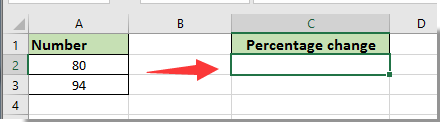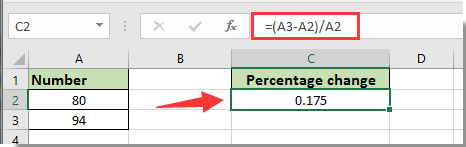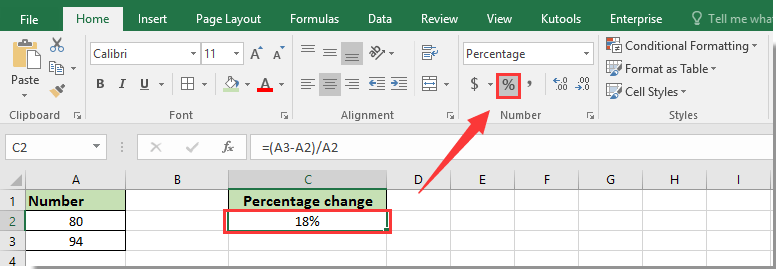## How to calculate percentage change or difference between two numbers in Excel?

#### Calculate percentage change/difference between two numbers with formula

As below screenshot shown, you need to calculate the percentage change between new number 94 and old number 80. The formula =(new_value-old_value)/old_value can help you quickly calculate the percentage change between two numbers. Please do as follows.1. Select a blank cell for locating the calculated percentage change, then enter formula =(A3-A2)/A2 into the Formula Bar, and then press the Enter key. See screenshot:2. Keep selecting the result cell, then click the Percent Style button in the Number group under Home tab to format the cell as percentage. See screenshot:Now the percentage difference between two given numbers is calculated.

### Best Office Productivity Tools

 Popular Features: Find, Highlight or Identify Duplicates   |  Delete Blank Rows   |  Combine Columns or Cells without Losing Data   |   Round without Formula ... Super Lookup: Multiple Criteria VLookup  |   Multiple Value VLookup  |   VLookup Across Multiple Sheets   |   Fuzzy Lookup .... Advanced Drop-down List: Quickly Create Drop Down List   |  Dependent Drop Down List   |  Multi-select Drop Down List .... Column Manager: Add a Specific Number of Columns  |  Move Columns  |  Toggle Visibility Status of Hidden Columns  |  Compare Ranges & Columns ... Featured Features: Grid Focus   |  Design View   |   Big Formula Bar   |  Workbook & Sheet Manager   |  Resource Library (Auto Text)   |  Date Picker   |  Combine Worksheets   |  Encrypt/Decrypt Cells   |  Send Emails by List   |  Super Filter   |   Special Filter (filter bold/italic/strikethrough...) ... Top 15 Toolsets:  12 Text Tools (Add Text, Remove Characters, ...)   |   50+ Chart Types (Gantt Chart, ...)   |   40+ Practical Formulas (Calculate age based on birthday, ...)   |   19 Insertion Tools (Insert QR Code, Insert Picture from Path, ...)   |   12 Conversion Tools (Numbers to Words, Currency Conversion, ...)   |   7 Merge & Split Tools (Advanced Combine Rows, Split Cells, ...)   |   ... and more

Supercharge Your Excel Skills with Kutools for Excel, and Experience Efficiency Like Never Before. Kutools for Excel Offers Over 300 Advanced Features to Boost Productivity and Save Time.  Click Here to Get The Feature You Need The Most...#### Office Tab Brings Tabbed interface to Office, and Make Your Work Much Easier

• Enable tabbed editing and reading in Word, Excel, PowerPoint, Publisher, Access, Visio and Project.
• Open and create multiple documents in new tabs of the same window, rather than in new windows.
• Increases your productivity by 50%, and reduces hundreds of mouse clicks for you every day!Rated 5 out of 5 · 1 ratings
This comment was minimized by the moderator on the site
((new value/old value)-1)*100 For % change
This comment was minimized by the moderator on the site
=((new value/old value)-1)*100 For % change
This comment was minimized by the moderator on the site
doesnt work
This comment was minimized by the moderator on the site
it is possible to calculate three values with the percentage change?
Rated 5 out of 5
This comment was minimized by the moderator on the site
Hi Edi Silva,
You can try this formula: =(first value-second value)/(third value-second value)
This comment was minimized by the moderator on the site
I have 0 in A1, 5 in B1, increase in percentage should be 500%. However with the given formula (B1-A1)/A1 = (0-500)/0 gives me a #DIV/0! error.In case if i apply =IF(A2<>0,(A3-A2)/A2,"0"), it return s me "0". neither of them helps. Any solution to this???
This comment was minimized by the moderator on the site
In case anyone still needs an answer for this problem, use this =IF(A1<>0, (B1-A1)/A1, (B1-A1))
using the percent style format will make (B1-A1) in this case (5-0) = 5 become 500%.
This comment was minimized by the moderator on the site
Hey Stanley,

Thanks for the formula!
Would you mind explaining the formula, please?
This comment was minimized by the moderator on the site
SMH SMH SMH SMH SMH
This comment was minimized by the moderator on the site
I hope you're not that dumb, dividing by 0
This comment was minimized by the moderator on the site
Any help for picking up value only it falls between 85% to 99% else blank.
Such as A is 10 and in B if we put any number , and in C it show the value only if it is fals between 85% to 99% of A
This comment was minimized by the moderator on the site
When a cell is blank, and another cell Has a number, how do you
This comment was minimized by the moderator on the site
Hello, Need help on how to write an formula with this situation.
So i have a report card design to show the grade of each performance of the work output. I set a standard for a specific task in amount of 350. However, based on available volume, not all the month output can be reach to 350. Therefore, we calculated that for this month, the person can achieve their 100% goal by just reaching 174. However, that person can go beyond that number to reach 350. What i want to do here is that our grade level is showing below. If they achieve 174, they would get a grade level B which is between 95% to 105%. But in order to get to maximum 120% of grade A+, the person will need to reach 350. This is where i stuck with. So i know that if a person reaches 180, he/she would get somewhere 102 or 103%. But i dont know how to calculate this and be able to set a maximum 120% between the number of 174 and 350

So right now if the number double from 174 to 348, the % is 200%.... but i need to set this maximum 120% only. How can i do this?

0.00% -

40.00% E-

45.00% E

50.00% E+

55.00% D-

65.00% D

70.00% D+

75.00% C-

80.00% C

85.00% C+

90.00% B-

95.00% B

105.00% B+

110.00% A-

115.00% A

120.00% A+
This comment was minimized by the moderator on the site
Percentage 'change' and percentage 'difference' are two different things. This formula represents percentage change, for example if you are are comparing values of the same statistic over time (e.g. product sales this year compared to product sales last year). Percentage difference is different. Difference can be used when comparing two different statistics to each other (e.g. Mary's annual sales compared to Martha's annual sales). Mary didn't have an increase or decrease on Martha's sales over the same time period, they were just different. To calculate percentage difference you take the absolute value of the difference between two numbers. Then divide it by the average of those two numbers and then multiple by 100 to get the percentage. For example, if the two values are 40 and 60. The difference is 20, ignoring whether it's positive or negative. The average is 50. 20/50 = .4 X 100 = 40%. The percentage increase between these two numbers, if it is an increase, would be 50%. The percentage decrease would be 33%. Close, but all very different.
This comment was minimized by the moderator on the site
1.The percentage increase between these two numbers, if it is an increase, would be 50%. The percentage decrease would be 33%. Close, but all very different.....  How did you get the 50 and the 33?2. If you say Marias YOY is 8% and Martha's YOY is -25%, how do you calculate the difference between those two YOYs?
This comment was minimized by the moderator on the site
The formula that works in all cases is "=(new_value - old_value) / abs(old_value)"
This comment was minimized by the moderator on the site
How can I chnage regular percent change into Year over Year PCH?
There are no comments posted here yet